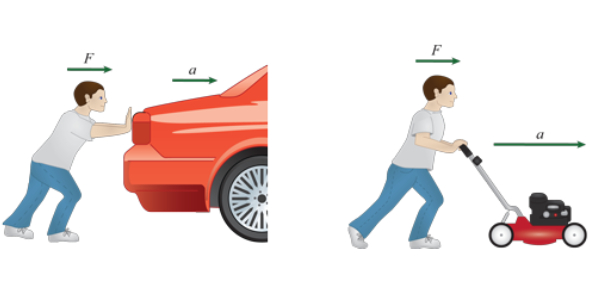# Newton's 2nd Law Exam Questions! Quiz

10 Questions | Attempts: 6529
ShareSettings2ND LAW physics.

• 1.
Compared to a 1kg block of solid iron, a 2kg block of solid iron has twice as much:
• A.

Inertia

• B.

Mass

• C.

Volume

• D.

All

• E.

None

• 2.
An object weighs 30N on earth. A second object weighs 30N on the moon. Which the greater mass? (Note: due to its size, the moon has less gravity than the earth.)
• A.

Object on moon

• B.

Object on earth

• C.

Same mass

• D.

Depends on air resistance

• 3.
One object had twice as much mass as another object. The first object also has twice as much:
• A.

Volume

• B.

Gravitational acceleration

• C.

Inertia

• D.

Velocity

• 4.
An object is propelled along a straight-line path by force. If the net force were doubled, its acceleration would:
• A.

Halve

• B.

Double

• C.

• D.

Stay the same

• 5.
The force of friction on a sliding object is 10 newtons. The applied force needed to maintain a constant velocity is:
• A.

Less than 10N

• B.

More than 10N

• C.

10N

• 6.
A 10N falling object encounters 4N of air resistance. The net force on the object is:
• A.

4N

• B.

0N

• C.

6N

• D.

10N

• E.

None of the above

• 7.
Which of the following has zero acceleration? An object
• A.

Moving at a constant velocity

• B.

At rest

• C.

At equilibrium

• D.

All of the above

• 8.
A 10kg block with an initial velocity of 10m/s slides 10m across a horizontal surface and comes to rest. It takes the block 2 seconds to stop. The stopping force acting on the block is about:
• A.

5N

• B.

10N

• C.

25N

• D.

50N

• 9.
A 10kg block is pushed across a horizontal surface with a horizontal force of 20N against a friction force of 10N. The acceleration of the block in meters per second per second is:
• A.

1

• B.

5

• C.

2

• D.

10

• 10.
A hockey puck is set in motion across a frozen pond. If ice friction and air resistance are neglected, the force required to keep the puck sliding at constant velocity is:
• A.

Equal to the weight of the puck

• B.

The weight of the puck divided by the mass of the puck

• C.

The mass of the puck multiplied by 9.8 m/s

• D.

Zero newtons

## Related TopicsBack to top
×

Wait!
Here's an interesting quiz for you.